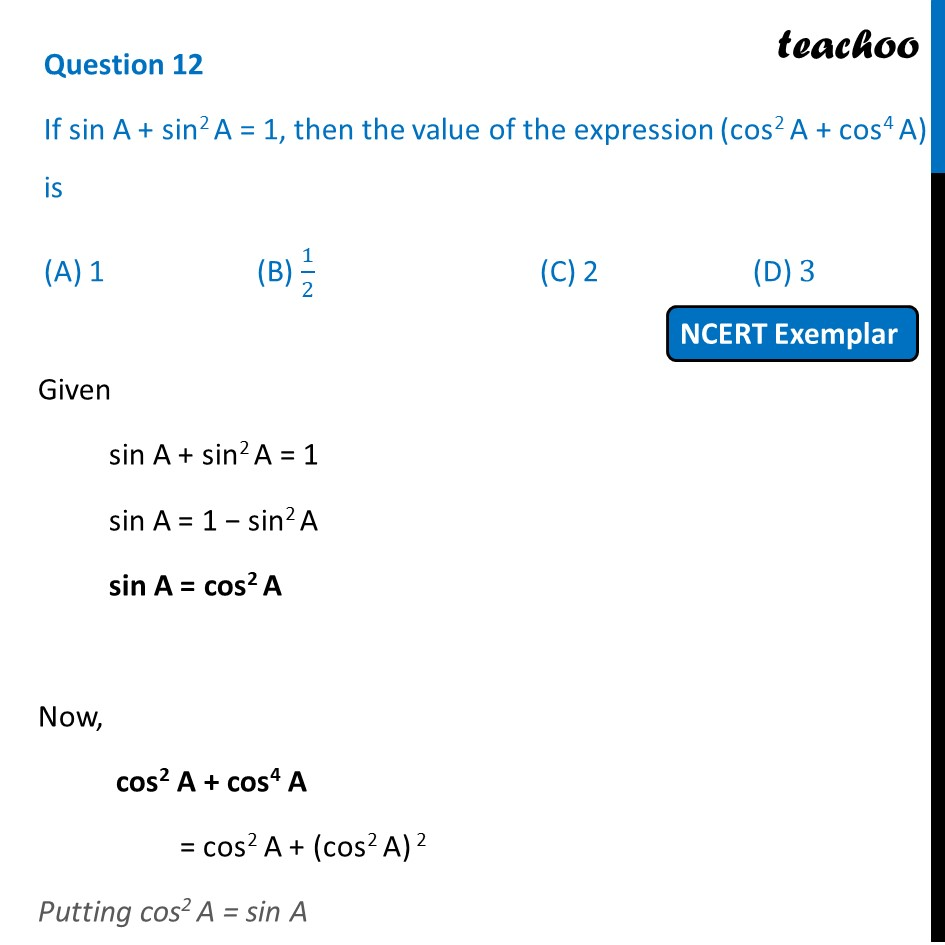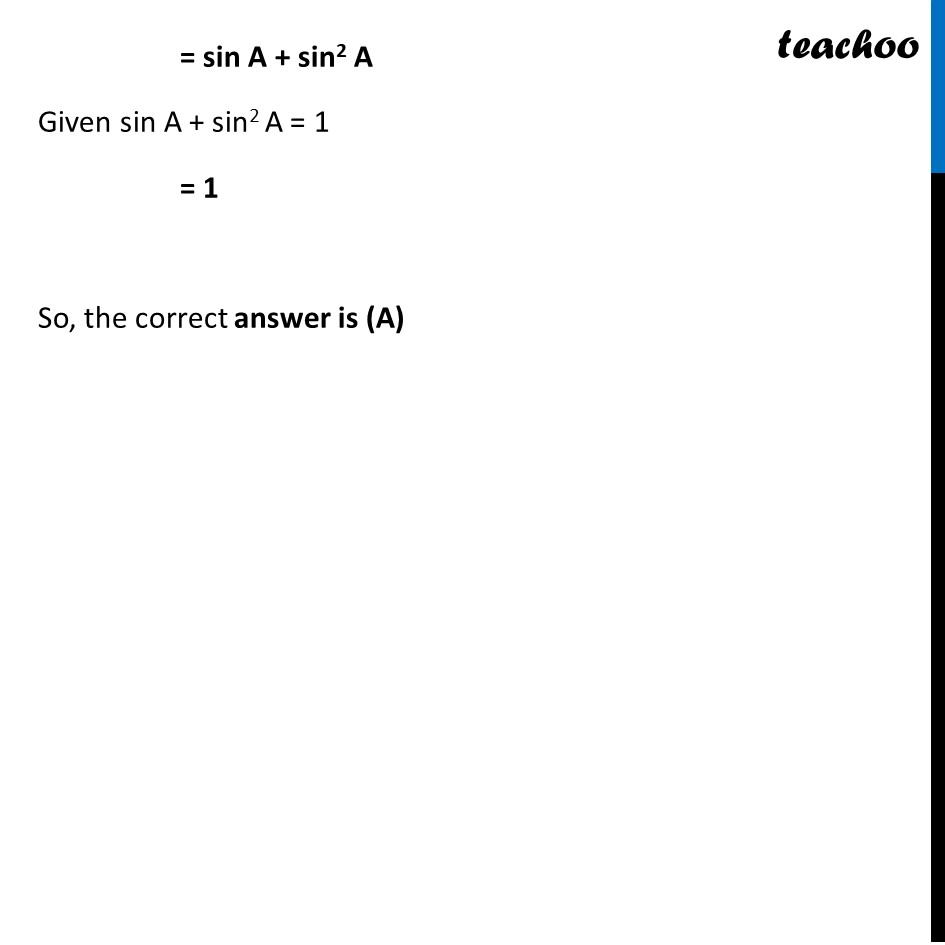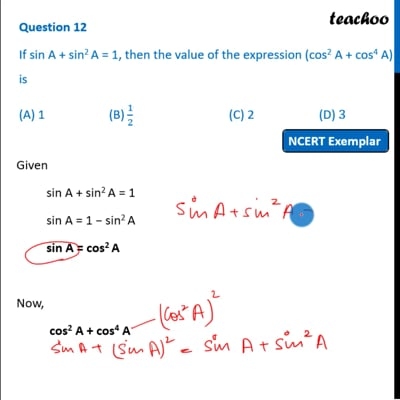NCERT Exemplar - MCQ

Chapter 8 Class 10 Introduction to Trignometry
Serial order wise

## (A) 1  (B) 1/2   (C) 2   (D) 3This video is only available for Teachoo black users

Introducing your new favourite teacher - Teachoo Black, at only ₹83 per month

### Transcript

Question 12 If sin A + sin2 A = 1, then the value of the expression (cos2 A + cos4 A) is (A) 1 (B) 1/2 (C) 2 (D) 3 Given sin A + sin2 A = 1 sin A = 1 − sin2 A sin A = cos2 A Now, cos2 A + cos4 A = cos2 A + (cos2 A) 2 Putting cos2 A = sin A = sin A + sin2 A Given sin A + sin2 A = 1 = 1 So, the correct answer is (A)